2021 NFL Defensive Rookie of the Year Odds
+225
2.25 to 1Micah Parsons
19.2% implied probability

+750
7.5 to 1Odafe Oweh
7.3% implied probability

+1000
10 to 1Patrick Surtain
5.7% implied probability

+1000
10 to 1Greg Rousseau
5.7% implied probability

+1000
10 to 1Jamin Davis
5.7% implied probability

+1000
10 to 1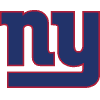Azeez Ojulari
5.7% implied probability

+1200
12 to 1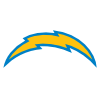Asante Samuel
4.8% implied probability

+1200
12 to 1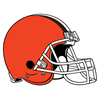Jeremiah Owusu-Koramoah
4.8% implied probability

+1600
16 to 1Joe Tryon-Shoyinka
3.7% implied probability

+2500
25 to 1Osa Odighizuwa
2.4% implied probability

+3000
30 to 1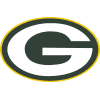Eric Stokes
2.0% implied probability

+4000
40 to 1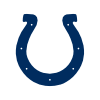Kwity Paye
1.5% implied probability

+4000
40 to 1Zaven Collins
1.5% implied probability

+5000
50 to 1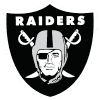Trevon Moehrig
1.2% implied probability

+5000
50 to 1Jabril Cox
1.2% implied probability

+5000
50 to 1Greg Newsome
1.2% implied probability

+5000
50 to 1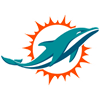Jaelan Phillips
1.2% implied probability

+10000
100 to 1Justin Hilliard
0.6% implied probability

+10000
100 to 1Nate Hobbs
0.6% implied probability

+10000
100 to 1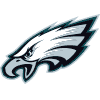Milton Williams
0.6% implied probability

+10000
100 to 1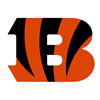Cameron Sample
0.6% implied probability

+10000
100 to 1Daviyon Nixon
0.6% implied probability

+10000
100 to 1Tyson Campbell
0.6% implied probability

+10000
100 to 1Jamar Johnson
0.6% implied probability

+10000
100 to 1Bobby Brown
0.6% implied probability

+10000
100 to 1Tommy Togiai
0.6% implied probability

+10000
100 to 1Hamsah Nasirildeen
0.6% implied probability

+10000
100 to 1Ar'Darius Washington
0.6% implied probability

+10000
100 to 1Payton Turner
0.6% implied probability

+10000
100 to 1Divine Deablo
0.6% implied probability

+10000
100 to 1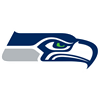Tre Brown
0.6% implied probability

+10000
100 to 1Benjamin St-Juste
0.6% implied probability

+10000
100 to 1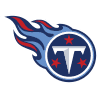Rashad Weaver
0.6% implied probability

+10000
100 to 1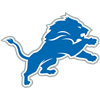Derrick Barnes
0.6% implied probability

+10000
100 to 1Elijah Molden
0.6% implied probability

+10000
100 to 1Caleb Farley
0.6% implied probability

+10000
100 to 1Tyler Shelvin
0.6% implied probability

+10000
100 to 1Ifeatu Melifonwu
0.6% implied probability

+10000
100 to 1Joseph Ossai
0.6% implied probability

+10000
100 to 1Jevon Holland
0.6% implied probability

+10000
100 to 1Jaycee Horn
0.6% implied probability

+10000
100 to 1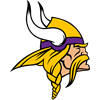Chazz Surratt
0.6% implied probability

+10000
100 to 1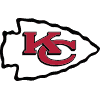Nick Bolton
0.6% implied probability

+10000
100 to 1Christian Barmore
0.6% implied probability

+10000
100 to 1Baron Browning
0.6% implied probability

+10000
100 to 1Aaron Robinson
0.6% implied probability

+10000
100 to 1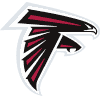Richie Grant
0.6% implied probability

+10000
100 to 1Kelvin Joseph
0.6% implied probability

+10000
100 to 1Carlos Basham
0.6% implied probability

+10000
100 to 1Ronnie Perkins
0.6% implied probability

+10000
100 to 1Pete Werner
0.6% implied probability

+10000
100 to 1Tay Gowan
0.6% implied probability

+10000
100 to 1Thomas Graham
0.6% implied probability

+10000
100 to 1Levi Onwuzurike
0.6% implied probability

+10000
100 to 1Andre Cisco
0.6% implied probability

+10000
100 to 1Marvin Wilson
0.6% implied probability

+10000
100 to 1Dayo Odeyingbo
0.6% implied probability

+10000
100 to 1Alim McNeill
0.6% implied probability